International
Tables for
Crystallography
Volume H
Powder diffraction
Edited by C. J. Gilmore, J. A. Kaduk and H. Schenk

International Tables for Crystallography (2018). Vol. H, ch. 3.9, pp. 348-350

## Section 3.9.3.6. Rietveld-based QPA

I. C. Madsen,a* N. V. Y. Scarlett,a R. Kleebergb and K. Knorrc

aCSIRO Mineral Resources, Private Bag 10, Clayton South 3169, Victoria, Australia,bTU Bergakademie Freiberg, Institut für Mineralogie, Brennhausgasse 14, Freiberg, D-09596, Germany, and cBruker AXS GmbH, Oestliche Rheinbrückenstr. 49, 76187 Karlsruhe, Germany
Correspondence e-mail:  ian.madsen@csiro.au

#### 3.9.3.6. Rietveld-based QPA

| top | pdf |

The advent of the Rietveld method (Rietveld, 1969) and its extension into the field of QPA (Bish & Howard, 1988; Hill, 1983; Hill & Howard, 1987; O'Connor & Raven, 1988; Taylor, 1991) has brought some significant benefits when compared with the conventional single-peak and pattern-addition methods. Recent surveys (Madsen et al., 2001; Scarlett et al., 2002) show that the majority of participants, greater than 75%, use a Rietveld-based approach for QPA. The benefits derive from (Hill, 1991; Kaduk, 2000):

 (i) The use of the entire diffraction pattern. Depending on the 2θ range of the data and the crystallography of the component phases, this may involve hundreds or thousands of reflections rather than the few peaks in conventional methods. This helps to minimize the impact of some systematic sample-related effects such as preferred orientation and extinction. (ii) The ability to accurately deconvolute overlapping peaks to extract the component intensities, thus allowing more complex patterns to be analysed. The development of fundamental-parameters models (Bergmann et al., 1998, 2000; Cheary & Coelho, 1992; Cheary et al., 2004), which aim to distinguish instrument from sample contributions to the diffraction pattern, minimizes the number of profile parameters that need to be refined, further enhancing this profile-fitting step. (iii) Refinement of the crystal structure, when supported by the data, to minimize differences between the intensities in the calculated and observed patterns. This brings additional information such as systematic changes in structure parameters from published data. (iv) The ability to model some remaining systematic effects such as preferred orientation or anisotropic crystallite size/strain peak broadening.

The Rietveld method uses a least-squares procedure to minimize the difference between a calculated pattern and the measured data. The calculated pattern is derived from a model containing crystal-structure information for each phase included in the analysis, convoluted with expressions describing peak shape and width, along with functions to correct systematic variances such as preferred orientation. The calculated pattern is multiplied by an overall scaling factor which may be equated to the peak intensities (Iiα) considered by the single-peak methods. The Rietveld scale factor for phase α, Sα, can be defined as (Bish & Howard, 1988; Hill, 1991; Hill & Howard, 1987; O'Connor & Raven, 1988)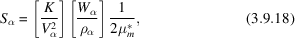where K is an 'experiment constant' used to put Wα on an absolute basis, and Vα, Wα and ρα are the volume of the unit cell, the weight fraction and the density for phase α, respectively.

Since equation (3.9.18)inherently contains the weight-fraction information, it can be rearranged to derive Wα: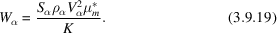O'Connor & Raven (1988) and Bish & Howard (1988) have shown that K is dependent only on the instrumental conditions and is independent of individual phase and overall sample-related parameters. Therefore a single measurement is sufficient to determine K for a given instrument configuration and set of data-collection conditions. Determination of K may be carried out by (i) a measurement of either a pure phase, or a phase of known proportion in a mixture, separately from the measurement of the actual unknown mixture, or (ii) using a phase that is present in the sample in a known amount. The value of K calculated in this way will be appropriate for the calibration of subsequent measurements as long as all instrumental and data-collection conditions remain the same as those used in its determination.

For each phase, the density ρα can be calculated from the published (or refined) crystal-structure information using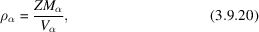where ZM is the mass of the unit-cell contents (Z is the number of formula units in the unit cell and M is the molecular mass of the formula unit) and V is the unit-cell volume.2

Substitution of equation (3.9.20)in equation (3.9.19)shows that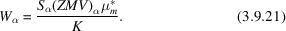In this context, (ZMV)α is the `phase constant' and can be calculated from published or refined crystal-structure information alone. It is worth noting that, if the crystal structure is refined as part of the analysis, ZMV is updated and hence becomes a dynamic phase constant.

The methodology embodied in equation (3.9.21)is important in many applications in that it produces, within the limits of experimental error, absolute phase abundances and is referred to hereafter as the external standard approach. While the use of a phase that already exists within the sample to determine K may be considered as an internal standard approach, in some applications, including in situ studies, that phase may be removed from the system through, for example, decomposition or dissolution. However, the value of K remains valid for subsequent determination of phase abundances provided that the instrumental and data-collection conditions do not change.

Equation (3.9.21)is directly applicable to the analysis of those phases for which detailed crystal-structure information is available. For phases where only a partial structure (for example, an indexed unit cell but no atom coordinates or site-occupation factors) is available, an empirical ZMV can be derived using mixtures of the phase of interest with known amounts of a well characterized standard (Scarlett & Madsen, 2006). QPA of phases with partial structure is also possible through the use of equation (3.9.19), but an estimate of the phase density, obtained through direct measurement, is required.

The limitations of the approach embodied in equations (3.9.19)and (3.9.21)derive from the need to (i) conduct a measurement of K and (ii) estimate the value of the mass absorption coefficient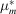for the sample(s) used to determine K, as well as for each sample of interest. However, similar to the earlier discussion about the single-peak methods,can be determined by direct measurement or calculation using equations (3.9.4)or (3.9.5), respectively. The benefits that can be derived from the extraction of the absolute, rather than relative, phase abundances, make it worth pursuing in many analytical situations. For example, in time-resolved studies where phases transform and material is lost in the course of reaction, the calculation of relative abundances summed to 100% may give a misleading impression of increased amounts of the remaining phases.

In some diffraction instruments, there may be decay in the incident-beam intensity during the course of measurement. This may happen on the timescale of months for a laboratory-based instrument owing to X-ray tube ageing, or on the scale of minutes at a synchrotron instrument where the storage-ring current is only refreshed once or twice per day. In this case, the change in incident intensity can be taken into account by incorporating an additional term into equation (3.9.21):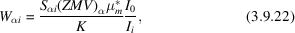where I0 and Ii are the incident beam intensities present during the determination of K and the collection of data set i, respectively.

The need to measure K, and measure or calculate, serves to increase the overall experimental difficulty and can be eliminated in ways analogous to those used in the single-peak methodology described earlier. For a simple two-phase mixture where both phases, α and β, are 100% crystalline, the sum of their weight fractions Wα and Wβ equals unity and can be expressed as (Bish & Howard, 1988)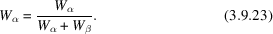Substitution of equation (3.9.21)for phases α and β in equation (3.9.23)results in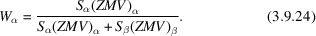Alternatively, in a multiphase sample, the addition of an internal standard s in known amount Ws and taking the ratio of equation (3.9.21)for analyte and standard phases provides the relationship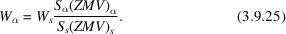The method embodied in equation (3.9.25)is analogous to the internal standard approach in equation (3.9.9)and also serves to produce absolute phase abundances Wα(abs). Once again, the benefit accruing from the use of absolute phase abundances is the ability to estimate the presence and amount of any amorphous and/or unidentified phases W(unk) through application of equations (3.9.16)and (3.9.17).

Hill & Howard (1987) and Bish & Howard (1988) have adapted the matrix-flushing method of Chung (1974a,b) to the Rietveld analysis context. By constraining the sum of the analysed weight fractions to the assumed concentration of the crystalline components (usually unity), the weight fraction of phase α in an n-phase mixture is given by the relationship3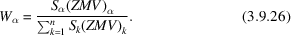The use of equation (3.9.26)in QPA again eliminates the need to measure the instrument calibration constant and estimate the sample mass absorption coefficient. However, the necessity of normalizing the sum of the analysed weight fractions to unity only produces the correct relative phase abundances. This approach is the most widely used in Rietveld-based QPA and is almost universally coded into Rietveld analysis programs. If the sample contains amorphous phases and/or minor amounts of unidentified crystalline phases, the analysed weight fractions will be overestimated. Where absolute phase abundances are required in, for example, the derivation of reaction mechanisms in in situ studies, then one of the methods that produces absolute phase abundances must be used.

### References

Bergmann, J., Friedel, P. & Kleeberg, R. (1998). Bgmn – a new fundamental parameters based Rietveld program for laboratory X-ray sources; its use in quantitative analysis and structure investigations. IUCr Commission on Powder Diffraction Newsletter, 20, 5–8.Google Scholar
Bergmann, J., Kleeberg, R., Haase, A. & Breidenstein, B. (2000). Advanced fundamental parameters model for improved profile analysis. Mater. Sci. Forum, 347–349, 303–308.Google Scholar
Bish, D. L. & Howard, S. A. (1988). Quantitative phase analysis using the Rietveld method. J. Appl. Cryst. 21, 86–91.Google Scholar
Cheary, R. W. & Coelho, A. (1992). A fundamental parameters approach to X-ray line-profile fitting. J. Appl. Cryst. 25, 109–121.Google Scholar
Cheary, R. W., Coelho, A. A. & Cline, J. P. (2004). Fundamental parameters line profile fitting in laboratory diffractometers. J. Res. Natl Inst. Stand. Technol. 109, 1–25.Google Scholar
Chung, F. H. (1974a). Quantitative interpretation of X-ray diffraction patterns of mixtures. I. Matrix-flushing method for quantitative multicomponent analysis. J. Appl. Cryst. 7, 519–525.Google Scholar
Chung, F. H. (1974b). Quantitative interpretation of X-ray diffraction patterns of mixtures. II. Adiabatic principle of X-ray diffraction analysis of mixtures. J. Appl. Cryst. 7, 526–531.Google Scholar
Hill, R. J. (1983). Calculated X-ray powder diffraction data for phases encountered in lead/acid battery plates. J. Power Sources, 9, 55–71.Google Scholar
Hill, R. J. (1991). Expanded use of the Rietveld method in studies of phase abundance in multiphase mixtures. Powder Diffr. 6, 74–77.Google Scholar
Hill, R. J. & Howard, C. J. (1987). Quantitative phase analysis from neutron powder diffraction data using the Rietveld method. J. Appl. Cryst. 20, 467–474.Google Scholar
Kaduk, J. A. (2000). In Industrial Applications of X-ray Diffraction, edited by F. H. Chung & D. K. Smith, pp. 207–253. New York: Marcel Dekker.Google Scholar
Madsen, I. C., Scarlett, N. V. Y., Cranswick, L. M. D. & Lwin, T. (2001). Outcomes of the International Union of Crystallography Commission on Powder Diffraction Round Robin on Quantitative Phase Analysis: samples 1a to 1h. J. Appl. Cryst. 34, 409–426.Google Scholar
O'Connor, B. H. & Raven, M. D. (1988). Application of the Rietveld refinement procedure in assaying powdered mixtures. Powder Diffr. 3, 2–6.Google Scholar
Rietveld, H. M. (1969). A profile refinement method for nuclear and magnetic structures. J. Appl. Cryst. 2, 65–71.Google Scholar
Scarlett, N. V. Y. & Madsen, I. C. (2006). Quantification of phases with partial or no known crystal structures. Powder Diffr. 21, 278–284.Google Scholar
Scarlett, N. V. Y., Madsen, I. C., Cranswick, L. M. D., Lwin, T., Groleau, E., Stephenson, G., Aylmore, M. & Agron-Olshina, N. (2002). Outcomes of the International Union of Crystallography Commission on Powder Diffraction Round Robin on Quantitative Phase Analysis: samples 2, 3, 4, synthetic bauxite, natural granodiorite and pharmaceuticals. J. Appl. Cryst. 35, 383–400.Google Scholar
Taylor, J. C. (1991). Computer programs for standardless quantitative analysis of minerals using the full powder diffraction profile. Powder Diffr. 6, 2–9.Google Scholar